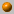##### Personal tools
You are here: Home Computational Geometry - Spring 2008
« December 2019 »
December
SuMoTuWeThFrSa
1234567
891011121314
15161718192021
22232425262728
293031

# Computational Geometry - Spring 2008

Instructor: Dan Halperin , danha AT post

Monday, 16:00--19:00
Schreiber 007

Office hours: Wednesday, 18:00--19:00
Schreiber 219

The course covers fundamental algorithms for solving geometric problems such as computing convex hulls, intersection of line segments, Voronoi diagrams, polygon triangulation, and linear programming in low dimensional space. We will also discuss several applications of geometric algorithms to solving problems in robotics, GIS (geographic information systems), computer graphics, and more.

### Bibliography:

The main textbook of the course is
Computational Geometry: Algorithms and Applications (CGAA), 2nd or 3rd edition
by M. de Berg, M. van Kreveld, M. Overmars, and O. Schwarzkopf.

A bibliographic list for the course

Since there is no grader (bodek) for the course, there will be no official assignments. The students are encouraged to solve the exercises at the end of each relevant chapter in the course's textbook. The grade in the final exam, to be held on Monday, July 21st, 2008, will be the grade in the course.

### Course Summary:

Below you'll find a very brief summary of what was covered in class during the semester. This should not be taken as a complete description of the course's contents.

For an outline of the course, see for example, the course summary in the 2006 computational geometry course .5.05.08
What is Computational Geometry? Course outline, motivation and techniques (to be continued). Naive convex hull algorithms (see Chapter 3 in O'Rourke's book). Orientation (Side-of-line) test.12.05.08
Convex hull computation continued; Line segment intersection

Gift wrapping. Graham's O(n log n) algorithm (Chapter 1 in CGAA). Lower bound for convex hull algorithms.

Output sensitive algorithm to compute the intersections of line segments: Bentley Ottmann's plane sweep (Chapter 2 in CGAA).19.05.08
The doubly-connected edge list (DCEL), and overlay of planar subdivisions (CGAA, Chapter 2).

The Douglas-Peuker algorithm for line simplification.26.05.08
(Gill Barequet, The Technion) Art Gallery, polygon triangulation (CGAA, Chapter 3).
The slides (zipped pdf file).2.06.08
The casting problem. Half plane intersection. Introduction to Linear Programming. A randomized algoriothm for LP in 2D (CGAA, Chapter 4).16.06.08
Linear Programming continued. The unbounded case in 2D; sketch of LP in 3D (CGAA, Chapter 4).
Orthogonal range search I: kd-trees. CGAA, Chapter 5.23.06.08
Orthogonal range search II: range-trees. Relaxing the general position assumption. Fractional cascading. CGAA, Chapter 5.
The video Objects that cannot be taken apart with two hands by Jack Snoeyink, from the 1993 Video Review in the 19th ACM Symposium on Computational Geometry.
Point location, introduction.
Point in polygon. How reliable are practical point-in-polygon strategies? by Stefan Schirra. To appear in the porceedings of the European Symposium on Algorithms (ESA) 2008.

Handouts, slides of Chapter 6.27.06.08 (Friday, the extra lesson by the "mitveh")
Point location in planar maps, trapezoidal maps, randomized incremental construction. CGAA, Chapter 6 (slide handouts above).30.06.08
Voronoi diagrams. CGAA, Chapter 7.

Handouts, slides of Chapter 7.07.07.08
Line arrangements and duality. CGAA, Chapter 8.

Handouts, slides of Chapter 8.14.07.08
Delaunay triangulations. CGAA, Chapter 9.

Handouts, slides of Chapter 9.
##### Document Actions